# Marginal effects at specific values or levels

This vignettes shows how to calculate marginal effects at specific values or levels for the terms of interest. It is recommended to read the general introduction first, if you haven’t done this yet.

The terms-argument not only defines the model terms of interest, but each model term can be limited to certain values. This allows to compute and plot marginal effects for (grouping) terms at specific values only, or to define values for the main effect of interest.

There are several options to define these values, which always should be placed in square brackets directly after the term name and can vary for each model term.

1. Concrete values are separated by a comma: terms = "c172code [1,3]". For factors, you could also use factor levels, e.g. terms = "Species [setosa,versicolor]".
2. Ranges are specified with a colon: terms = c("c12hour [30:80]", "c172code [1,3]"). This would plot all values from 30 to 80 for the variable c12hour.
3. Convenient shortcuts to calculate common values like mean +/- 1 SD (terms = "c12hour [meansd]"), quartiles (terms = "c12hour [quart]") or minumum and maximum values (terms = "c12hour [mixmax]"). See rprs_values() for the different options.
4. A function name. The function is then applied to all unique values of the indicated variable, e.g. terms = "hp [exp]".
5. Finally, if the first variable specified in terms is a numeric vector for which no specific values are given, a “pretty range” is calculated (see pretty_range()), to avoid memory allocation problems for vectors with many unique values. If a numeric vector is specified as second or third variable in term (i.e. if this vector represents a grouping structure), representative values (see rprs_values()) are chosen.

## Specific values and value range

library(ggeffects)
library(ggplot2)
data(efc)
fit <- lm(barthtot ~ c12hour + neg_c_7 + c161sex + c172code, data = efc)

mydf <- ggpredict(fit, terms = c("c12hour [30:80]", "c172code [1,3]"))
mydf
#>
#> # Predicted values for Total score BARTHEL INDEX
#> # x = average number of hours of care per week
#>
#> # low level of education
#>   x predicted conf.low conf.high
#>  30    67.147   64.037    70.257
#>  31    66.894   63.791    69.997
#>  32    66.641   63.545    69.736
#>  33    66.387   63.298    69.477
#>  34    66.134   63.051    69.217
#>  35    65.881   62.804    68.958
#>  36    65.628   62.556    68.699
#>  37    65.374   62.308    68.441
#>  38    65.121   62.059    68.183
#>  39    64.868   61.810    67.925
#>  ... and 41 more rows.
#>
#> # high level of education
#>   x predicted conf.low conf.high
#>  30    68.584   65.417    71.751
#>  31    68.331   65.165    71.497
#>  32    68.077   64.912    71.243
#>  33    67.824   64.660    70.989
#>  34    67.571   64.406    70.735
#>  35    67.318   64.153    70.482
#>  36    67.064   63.899    70.230
#>  37    66.811   63.645    69.977
#>  38    66.558   63.390    69.725
#>  39    66.304   63.135    69.474
#>  ... and 41 more rows.
#>
#> * neg_c_7 = 11.84
#> * c161sex = 1.76
ggplot(mydf, aes(x, predicted, colour = group)) + geom_line()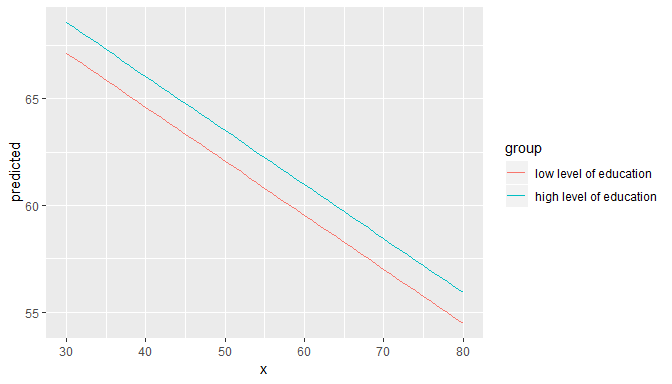Defining value ranges is especially useful when variables are, for instance, log-transformed. ggpredict() then typically only uses the range of the log-transformed variable, which is in most cases not what we want. In such situation, specify the range in the terms-argument.

data(mtcars)
mpg_model <- lm(mpg ~ log(hp), data = mtcars)

# x-values and predictions based on the log(hp)-values
ggpredict(mpg_model, "hp")
#>
#> # Predicted values for mpg
#> # x = hp
#>
#>    x predicted conf.low conf.high
#>  3.8    58.270   49.685    66.855
#>  4.0    57.718   49.255    66.181
#>  4.2    57.193   48.846    65.540
#>  4.4    56.692   48.456    64.928
#>  4.6    56.214   48.083    64.344
#>  4.8    55.756   47.726    63.785
#>  5.0    55.316   47.384    63.249
#>  5.2    54.894   47.055    62.733
#>  5.4    54.488   46.738    62.237
#>  5.6    54.096   46.433    61.760
#>  ... and 1 more rows.

# x-values and predictions based on hp-values from 50 to 150
ggpredict(mpg_model, "hp [50:150]")
#>
#> # Predicted values for mpg
#> # x = hp
#>
#>   x predicted conf.low conf.high
#>  50    30.531   27.947    33.115
#>  51    30.318   27.776    32.859
#>  52    30.109   27.609    32.608
#>  53    29.904   27.445    32.362
#>  54    29.702   27.283    32.121
#>  55    29.505   27.125    31.885
#>  56    29.311   26.969    31.653
#>  57    29.120   26.816    31.425
#>  58    28.933   26.665    31.202
#>  59    28.749   26.516    30.982
#>  ... and 91 more rows.

## Choosing representative values

Especially in situations where we have two continuous variables in interaction terms, or where the “grouping” variable is continuous, it is helpful to select representative values of the grouping variable - else, predictions would be made for too many groups, which is no longer helpful when interpreting marginal effects.

You can use

• "minmax": minimum and maximum values (lower and upper bounds) of the variable are used.
• "meansd": uses the mean value as well as one standard deviation below and above mean value.
• "zeromax": is similar to the "minmax" option, however, 0 is always used as minimum value. This may be useful for predictors that don’t have an empirical zero-value.
• "quart" calculates and uses the quartiles (lower, median and upper), including minimum and maximum value.
• "quart2" calculates and uses the quartiles (lower, median and upper), excluding minimum and maximum value.
• "all" takes all values of the vector.
data(efc)
# short variable label, for plot
attr(efc$c12hour, "label") <- "hours of care" fit <- lm(barthtot ~ c12hour * c161sex + neg_c_7, data = efc) mydf <- ggpredict(fit, terms = c("c161sex", "c12hour [meansd]")) plot(mydf)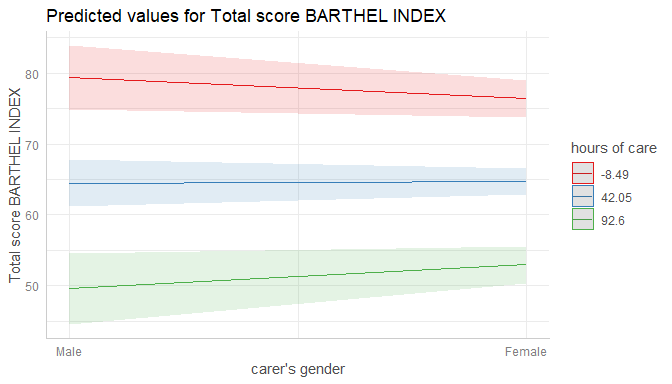mydf <- ggpredict(fit, terms = c("c161sex", "c12hour [quart]")) plot(mydf)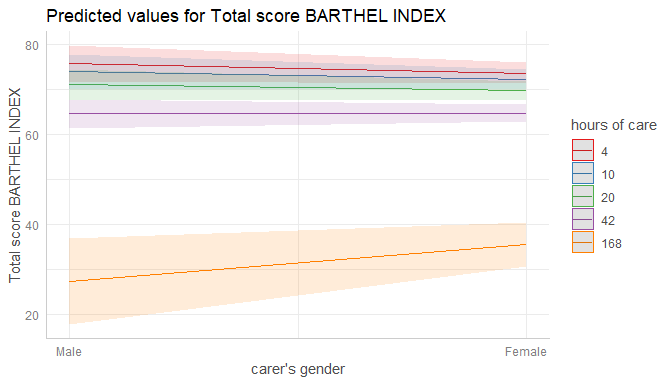## Transforming values with functions The brackets in the terms-argument also accept the name of a valid function, to (back-)transform predicted valued. In this example, an alternative would be to specify that values should be exponentiated, which is indicated by [exp] in the terms-argument: # x-values and predictions based on exponentiated hp-values ggpredict(mpg_model, "hp [exp]") #> #> # Predicted values for mpg #> # x = hp #> #> x predicted conf.low conf.high #> 52 30.109 27.609 32.608 #> 62 28.215 26.085 30.346 #> 65 27.707 25.671 29.742 #> 66 27.542 25.537 29.547 #> 91 24.085 22.652 25.517 #> 93 23.851 22.450 25.252 #> 95 23.622 22.251 24.993 #> 97 23.397 22.055 24.740 #> 105 22.544 21.296 23.793 #> 109 22.142 20.930 23.354 #> ... and 12 more rows. ## Pretty value ranges This section is intended to show some examples how the plotted output differs, depending on which value range is used. To see the difference in the “curvilinear” trend, we use a quadratic term on a standardized variable. library(sjmisc) data(efc) efc$c12hour <- std(efc\$c12hour)
m <- lm(barthtot ~ c12hour + I(c12hour^2) + neg_c_7 + c160age + c172code, data = efc)

me <- ggpredict(m, terms = "c12hour")
plot(me)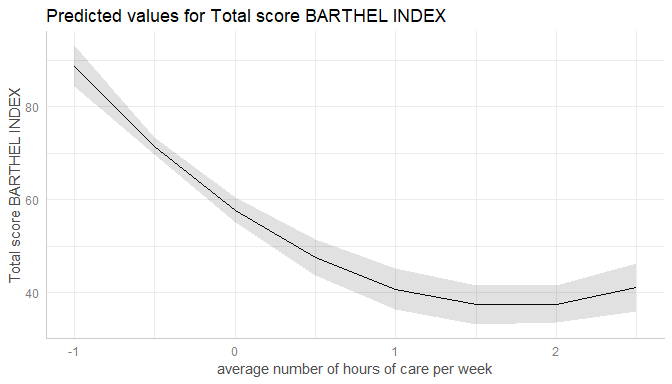ggpredict() “prettifies” the vector, resulting in a smaller set of unique values. This is less memory consuming and may be needed especially for more complex models.

You can turn off automatic “prettifying” by adding the "all"-shortcut to the terms-argument.

me <- ggpredict(m, terms = "c12hour [all]")
plot(me)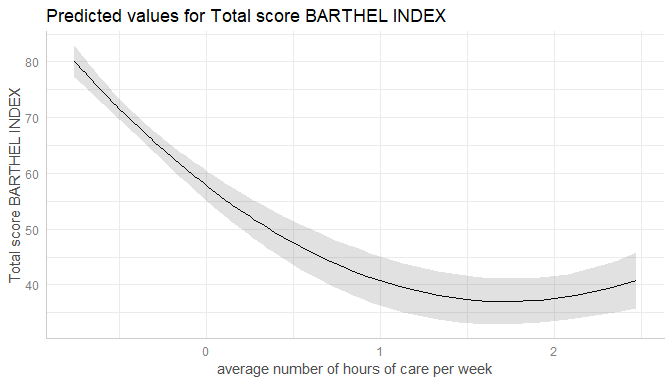This results in a smooth plot, as all values from the term of interest are taken into account.

## Marginal effects conditioned on specific values of the covariates

By default, the typical-argument determines the function that will be applied to the covariates to hold these terms at constant values. By default, this is the mean-value, but other options (like median or mode) are possible as well.

Use the condition-argument to define other values at which covariates should be held constant. condition requires a named vector, with the name indicating the covariate.

data(mtcars)
mpg_model <- lm(mpg ~ log(hp) + disp, data = mtcars)

# "disp" is hold constant at its mean
ggpredict(mpg_model, "hp [exp]")
#>
#> # Predicted values for mpg
#> # x = hp
#>
#>    x predicted conf.low conf.high
#>   52    25.609   21.938    29.281
#>   62    24.566   21.532    27.600
#>   65    24.286   21.421    27.151
#>   66    24.195   21.384    27.006
#>   91    22.291   20.565    24.016
#>   93    22.162   20.503    23.821
#>   95    22.036   20.441    23.631
#>   97    21.912   20.378    23.446
#>  105    21.442   20.123    22.762
#>  109    21.221   19.989    22.452
#>  ... and 12 more rows.
#>
#> * disp = 230.72

# "disp" is hold constant at value 200
ggpredict(mpg_model, "hp [exp]", condition = c(disp = 200))
#>
#> # Predicted values for mpg
#> # x = hp
#>
#>    x predicted conf.low conf.high
#>   52    26.297   22.971    29.623
#>   62    25.254   22.555    27.953
#>   65    24.974   22.440    27.508
#>   66    24.883   22.402    27.364
#>   91    22.979   21.518    24.440
#>   93    22.850   21.447    24.253
#>   95    22.724   21.374    24.073
#>   97    22.600   21.300    23.900
#>  105    22.130   20.990    23.271
#>  109    21.909   20.822    22.995
#>  ... and 12 more rows.